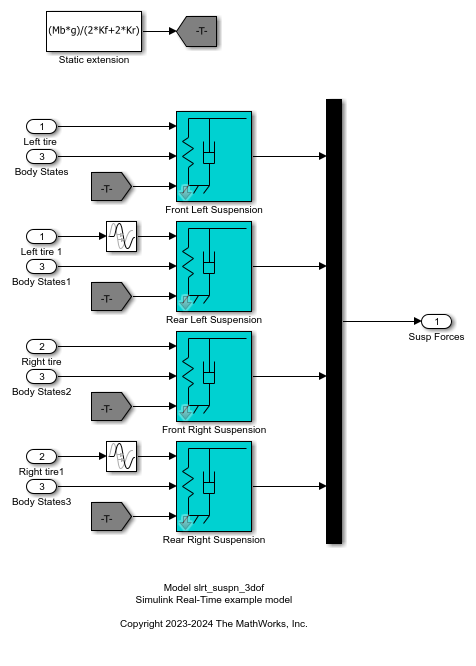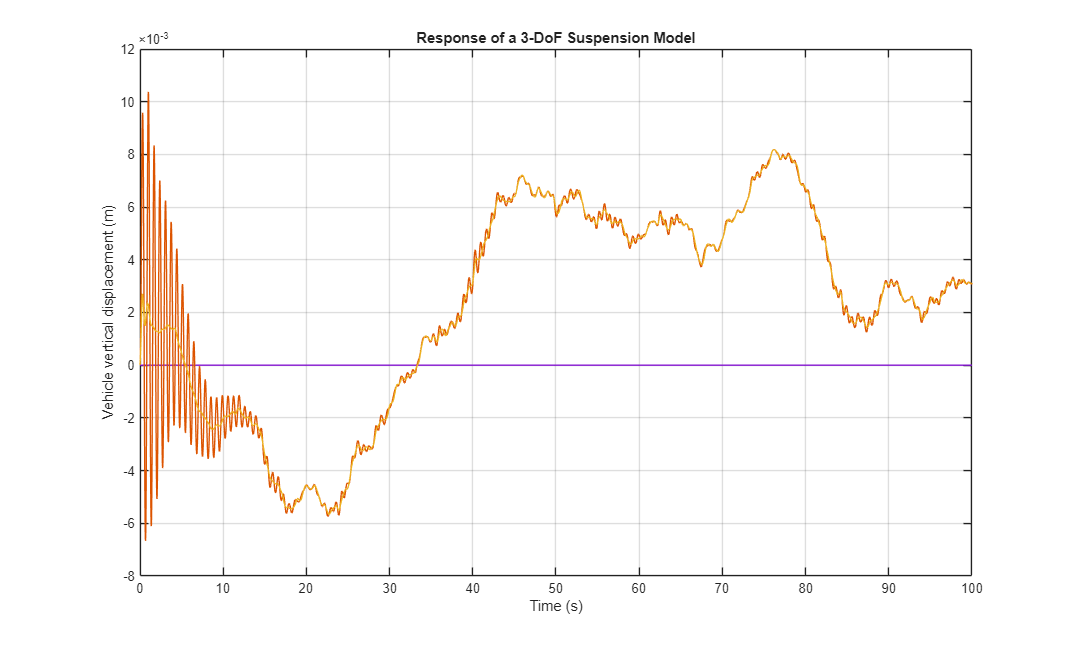# Data Logging with Simulation Data Inspector (SDI)

This example shows how to use a Simulink® Real-Time™ log of signal data and the Simulation Data Inspector. Signals are logged during model execution. At the end of the run, the Simulation Data Inspector interface displays the signal. This example show how to get the signals from the Simulation Data Inspector interface by using the command line.

Open the model `slrt_ex_soc_dist`. This model calibrates the control efforts through social distancing on an infectious disease outbreak.

Open the model.

```model = 'slrt_ex_soc_dist'; mdlOpen = 0; systems = find_system('type', 'block_diagram'); if all(~strcmp(model, systems)) mdlOpen = 1; open_system(fullfile(matlabroot,'toolbox','slrealtime','examples','slrt_ex_soc_dist.slx')); end ```• Configure for a non-Verbose build.

```set_param(model,'RTWVerbose','off'); evalc('slbuild(model)'); ```
• Close the model it is open.

```if (mdlOpen) bdclose(model); end ```

### Run Model to Evaluate Effects of No Social Distancing During Outbreak

Using the Simulink Real-Time object variable, `tg`, load and start the model, and modify model parameters.

```tg = slrealtime; load(tg,model); setparam(tg,'','soc_dist_level',1); setparam(tg,'','thresh_int_level',1); start(tg); while ~strcmp(tg.status,'stopped') pause(5); end stop(tg); ```

### Update Parameters and Re-evaluate Effect of Social Distancing During Outbreak

Using the Simulink Real-Time object variable, `tg`, load and start the model, and modify model parameters

```tg = slrealtime; load(tg,model); setparam(tg,'','soc_dist_level',0.2); setparam(tg,'','thresh_int_level',0.2); start(tg); while ~strcmp(tg.status,'stopped') pause(5); end stop(tg); ```

### Display Signals in Simulation Data Inspector

To view the plotted signal data, open the Simulation Data Inspector.

```Simulink.sdi.view ```

### Retrieve and Plot Signal Data from Simulation Data Inspector

You can also retrieve the signal data from the SDI and plot the data by using these commands.

• Get all the runs

• Get the run information

• Get the signal.

• Get the signal objects.

• Take only infectious group values.

• Plot the signals.

The result shows that social distancing can reduce the number of hospitalized people

```runIds = Simulink.sdi.getAllRunIDs(); for i = 1:length(runIds) run = Simulink.sdi.getRun(runIds(i)); signalID = run.getSignalIDsByName('hospitalized'); if ~isempty(signalID) signalObj = Simulink.sdi.getSignal(signalID); signalArray(:,i) = signalObj.Values(:,1).Data; timeValues = 100*(signalObj.Values(:,1).Time); plot(timeValues,signalArray); drawnow; end end grid on; xlabel('Time in days'); ylabel('hospitalized people'); ```